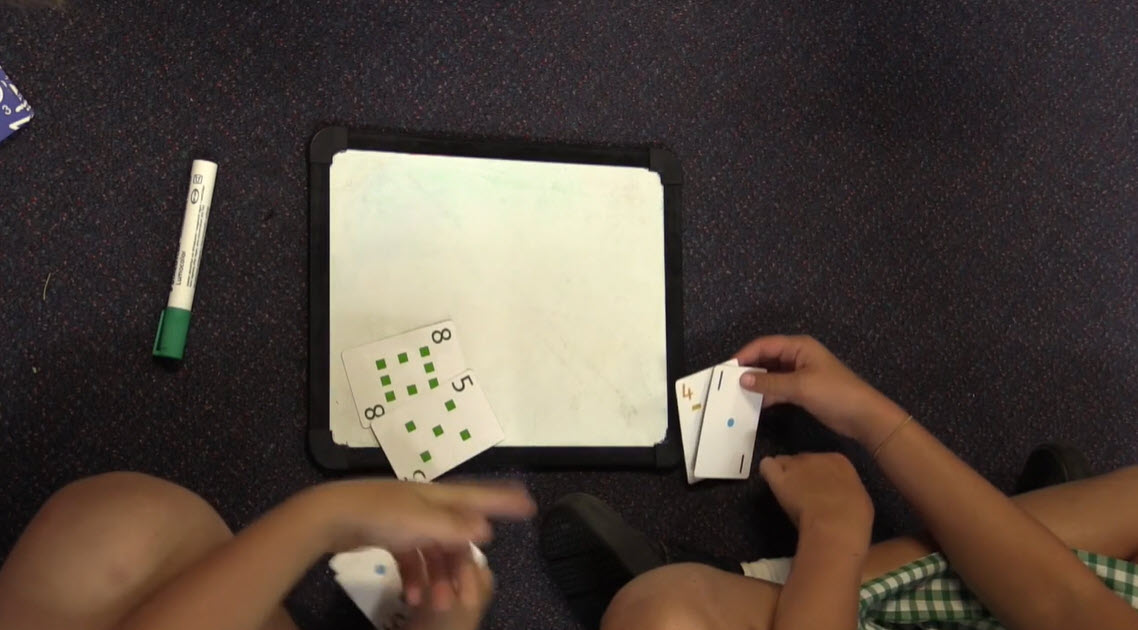Go to website

# Closest to 100

Uses number cards 1 to 9 to play a game where the goal for each team is to get as close as possible to a total of 100. A cumulative total of their difference to 100 is kept throughout. Addition and subtraction is used strategically and understanding of place value is involved as players move up towards 100.

Year level(s) Year 1, Year 2, Year 3
Audience Student, Teacher
Format Video
Keywords games, addition, subtraction, place value, fluency, reasoning, understanding, explicit teaching

## Curriculum alignment

Curriculum connections Numeracy
Strand and focus Number, Build understanding
Topics Place value, Addition and subtraction
AC: Mathematics (V9.0) content descriptions
AC9M1N04

Add and subtract numbers within 20, using physical and virtual materials, part-part-whole knowledge to 10 and a variety of calculation strategies

AC9M3A01
Recognise and explain the connection between addition and subtraction as inverse operations, apply to partition numbers and find unknown values in number sentences

AC9M2N04
Add and subtract one- and two-digit numbers, representing problems using number sentences and solve using part-part-whole reasoning and a variety of calculation strategies

Numeracy progression Additive strategies (P6, P7, P8)
Number and place value (P3, P5)
Number patterns and algebraic thinking (P3)